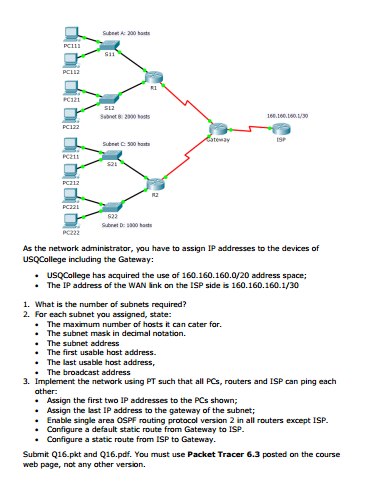# Answered! As the network administrator, you have to assign IP addresses to the devices of USQCollege including the…Question 1:

The address of the network formed by USQCollege is 160.160.160.0/20 and the subnet mask is (11111111.11111111.11110000.00000000)2 = (255.255.240.0)10
The first byte of the network address( i.e 160) indicates that it is a class B address( as first byte of class B address ranges from 128 to 191). The default subnet mask of class B is 16 or 255.255.0.0 .Therefore we need extra 20 -16 = 4 1’s to meet the requirement of the subnet as mentioned in the question.
Therefore total number of subnet = 2x (where x is the number of borrowed bits or number of 1’s)=24 = 16
Therefore the number of subnets required is 16.

Don't use plagiarized sources. Get Your Custom Essay on
Answered! As the network administrator, you have to assign IP addresses to the devices of USQCollege including the…
GET AN ESSAY WRITTEN FOR YOU FROM AS LOW AS \$13/PAGE

Question 2:

The maximum number of hosts it can cater for:

The subnet mask for the network is 20.
Therefore maximum number of hosts in this network can be computed as
log2 n = 32(total number of bits in IPv4 address) – subnet mask, where n is the total number of hosts in each subnet.
log2 n = 32-20 = 12
Therefore n = 212 = 4096.

Note that in each subnet there are two addresses kept reserved: one for subnet address and another for broadcast address. Therefore total number of usable addresses in each subnet are 4096 – 2 = 4094.Therefore maximum number of hosts it can refer = 4094.

The subnet mask in decimal notation:

As explained earlier, the subnet mask is 20. Therefore in binary the subnet mask is (11111111.11111111.11110000.00000000)2 (total number of 1’s is 20) = (255.255.240.0)10

Therefore the subnet mask in decimal notation is 255.255.240.0

The first address is considered as the subnet address. The first address of a subnet can be found by setting (32 – subnet mask) number of last bits of the address (in binary notation) to 0.

The IP address = 160.160.160.0/20 (last 32 -20 = 12 bits are set to 0 which results 10100000.10100000.10100000.00000000).

As I mentioned before that 2 addresses are kept reserved for a subnet: one is the subnet address and another is the broadcast address.

160.160.160.0 is used for subnet address. Therefore the first usable address is 160.160.160.1 (or 10100000.10100000.10100000.00000001)